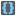• # This recursion gave me error

## Question related to missionBrackets

Hi,

I tried to solve this task with recursion with the following code (forgive if it's not Pythonic, I'm learning it):

```BRACKETS={'{':'}', '[':']','(':')'}

def analyzeString(string, bracketsOpened):

if not string: # if the string is finished, return True if there are no brackets to match, False otherwise
return not bracketsOpened

if not ( any(string == x for x in BRACKETS.keys()) or any(string == x for x in BRACKETS.values()) ):   #if the current character is NOT a bracket, move on
return analyzeString(string[1:],bracketsOpened)

if any(string == x for x in BRACKETS.keys()):   #if the current char is a left bracket, memorize in the LIFO and go on
bracketsOpened.append(string)
return analyzeString(string[1:],bracketsOpened)

if any(string == x for x in BRACKETS.values()) and not bracketsOpened:   #if the current char is a right bracket and there are no brackets in the LIFO, that's an error
return False

if string == BRACKETS[bracketsOpened[-1]] :    # if the bracket matches, that's all right, just go on
return analyzeString(string[1:],bracketsOpened[:-1])

#if you are here, the char is a bracket that doesn't matches with the last bracket in the LIFO, that's an error
return False

def checkio(string):
return analyzeString(string,[])
```

In checking whether this code solved the problem, it gave me error because it ended recursion memory (I think) for the case with a lot of brackets (so I needed to solve this task using a for loop).

I was wondering

1. if this code is correct overall and the problem is "only" memory

2. if this is Pythonic enough :D and

3. in case mine is incorrect, how could I solve the task recursively.

Thank you!